# Arithmetic Progressions - Olympiad Level MCQ, Class 10 Mathematics

## 25 Questions MCQ Test Olympiad Preparation for Class 10 | Arithmetic Progressions - Olympiad Level MCQ, Class 10 Mathematics

Description
Attempt Arithmetic Progressions - Olympiad Level MCQ, Class 10 Mathematics | 25 questions in 25 minutes | Mock test for Class 10 preparation | Free important questions MCQ to study Olympiad Preparation for Class 10 for Class 10 Exam | Download free PDF with solutions
QUESTION: 1

Solution:
QUESTION: 2

### 15th term of the A.P., x – 7, x – 2, x + 3,.... is

Solution:

[Soln: an = a + (n - 1)× d
where d = x – 2 - x + 7 = 5, a = x - 7
⇒a15 =(x - 7) + (15 - 1) ×5 = (x - 7) + 14 ×5
⇒a15 = x - 7 + 70 = x + 63]

QUESTION: 3

### The sum of first 24 terms of an A.P. a1, a2, a3,...; if it is known that a1 + a5 + a10 + a15 + a20 + a24 = 225, is equal to :

Solution:
QUESTION: 4

A student read common difference of an AP is – 2 instead of 2 and got the sum of first five terms as – 5. The actual sum of first five terms is :

Solution:
QUESTION: 5

The sum of n terms of two A.P's are in the ratio of (7n + 1) : (4n + 27). The ratio of their 11th terms is –

Solution:
QUESTION: 6

If 12 + 22 + 32 + ..... n2 = 1015, then the value of n is :

Solution:
QUESTION: 7

The sum of the series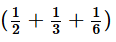+ ... upto 9 terms is :

Solution:
QUESTION: 8

The sum of first n odd natural numbers is :

Solution:
QUESTION: 9

If the roots of the equation x3 – 12x2 + 39x – 28 = 0 are in A.P., then their common difference will be :

Solution:
QUESTION: 10

If A.M between two numbers is 5 and their G.M. is 4, then their H.M. is :

Solution:
QUESTION: 11

If A is the single A.M. between two numbers a and b and S is the sum of n A.M.'s between them, then S/A depends upon :

Solution:
Given, A=a+b/2--------------------eq-1
we know that , s= n/2(a+l)
here,a=a,l=b
so, s=n/2(a+b)---------------------------eq-2
so, s=n[a+b/2]
A=a+b/2
equate 1&2
then , S/n=A
S/A=n
QUESTION: 12

If the A.M. between the roots of a quadratic equation is 8 and the G.M. is 5, then the equation is :

Solution:
QUESTION: 13

If c is the harmonic mean between a and b, then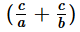is equal to :

Solution:
QUESTION: 14

If a,b,c,d,e,f are in A.P. then e–c is equal to :

Solution:
QUESTION: 15

20th term of the series :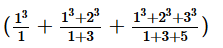+ ......... is :

Solution:
QUESTION: 16

If the value of 13 + 23 + 33 + ...+n3 = 2025, then the value of 1 + 2 + 3 +...+ n is :

Solution:
QUESTION: 17

If the value of 1 + 2 + 3 +... + n is 55, then the value of 13 + 23 + 33 + .... + n3  is :

Solution:
QUESTION: 18

The nth term of the series 1 +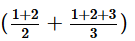+............... is :

Solution:
QUESTION: 19

12 + 1 + 22 + 2 + 32 + 3 + ... + n2 + n is equal to :

Solution:
QUESTION: 20

The next term of the sequence (1/4,1/36,1/144)   ............ is :

Solution:
QUESTION: 21

If the sum of first n natural numbers is one-fifth of the sum of their squares, then n equals :

Solution:
QUESTION: 22

The nth term of the series 1 + 3 + 6 + 10 + 15 + ... is :

Solution:
QUESTION: 23

The sum of the series 12 + 1 + 22 + 2 + 32 + 3 + ... + up to n terms is :

Solution:
QUESTION: 24

The nth term of the sequence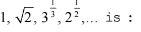Solution:
QUESTION: 25

The sum of n terms of the series (12 – 22) + (3– 42) + (52 – 62) +... is :

Solution:Use Code STAYHOME200 and get INR 200 additional OFF Use Coupon Code